# Introduction to precrec

#### 2023-01-08

The precrec package provides accurate computations of ROC and Precision-Recall curves.

## 1. Basic functions

The evalmod function calculates ROC and Precision-Recall curves and returns an S3 object.

library(precrec)

data(P10N10)

# Calculate ROC and Precision-Recall curves
sscurves <- evalmod(scores = P10N10$scores, labels = P10N10$labels)

### S3 generics

The R language specifies S3 objects and S3 generic functions as part of the most basic object-oriented system in R. The precrec package provides nine S3 generics for the S3 object created by the evalmod function.

S3 generic Package Description
print base Print the calculation results and the summary of the test data
as.data.frame base Convert a precrec object to a data frame
plot graphics Plot performance evaluation measures
autoplot ggplot2 Plot performance evaluation measures with ggplot2
fortify ggplot2 Prepare a data frame for ggplot2
auc precrec Make a data frame with AUC scores
part precrec Set partial curves and calculate AUC scores
pauc precrec Make a data frame with pAUC scores
auc_ci precrec Calculate confidence intervals of AUC scores

#### Example of the plot function

The plot function outputs ROC and Precision-Recall curves

# Show ROC and Precision-Recall plots
plot(sscurves)

# Show a Precision-Recall plot
plot(sscurves, "PRC")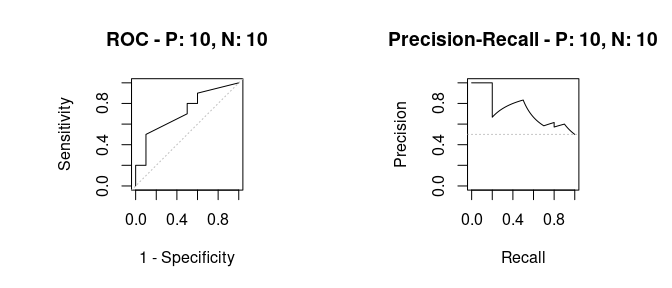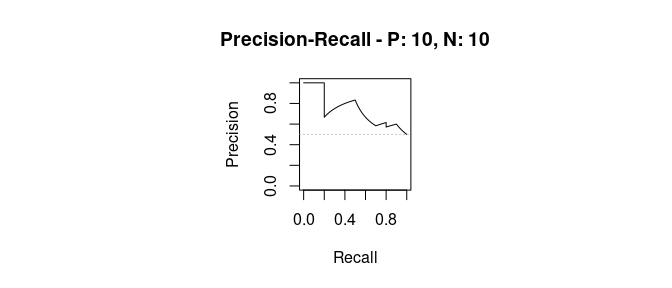#### Example of the autoplot function

The autoplot function outputs ROC and Precision-Recall curves by using the ggplot2 package.

# The ggplot2 package is required
library(ggplot2)

# Show ROC and Precision-Recall plots
autoplot(sscurves)

# Show a Precision-Recall plot
autoplot(sscurves, "PRC")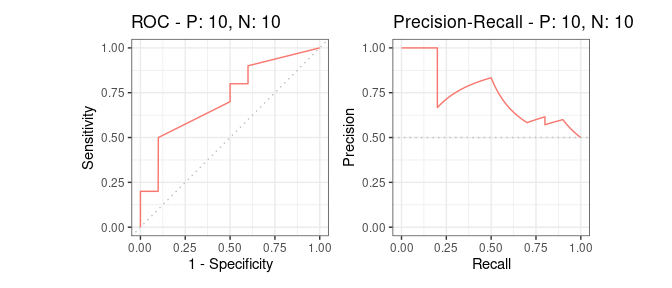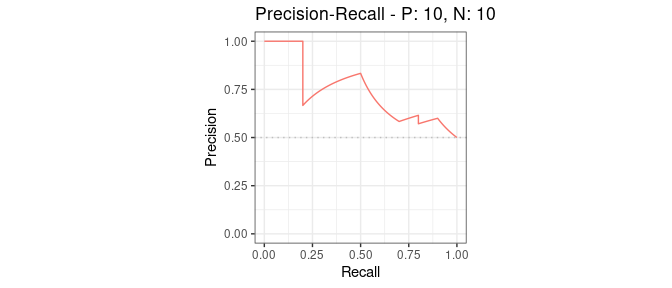Reduced supporting points make the plotting speed faster for large data sets.

# 5 data sets with 50000 positives and 50000 negatives
samp1 <- create_sim_samples(5, 50000, 50000)

# Calculate curves
eval1 <- evalmod(scores = samp1$scores, labels = samp1$labels)

# Reduced supporting points
system.time(autoplot(eval1))

# Full supporting points
system.time(autoplot(eval1, reduce_points = FALSE))
##    user  system elapsed
##   0.028   0.008   0.035
##    user  system elapsed
##   0.231   0.020   0.252

#### Example of the auc function

The auc function outputs a data frame with the AUC (Area Under the Curve) scores.

# Get a data frame with AUC scores
aucs <- auc(sscurves)

# Use knitr::kable to display the result in a table format
knitr::kable(aucs)
modnames dsids curvetypes aucs
m1 1 ROC 0.7200000
m1 1 PRC 0.7397716
# Get AUCs of Precision-Recall
aucs_prc <- subset(aucs, curvetypes == "PRC")
knitr::kable(aucs_prc)
modnames dsids curvetypes aucs
2 m1 1 PRC 0.7397716

#### Example of the as.data.frame function

The as.data.frame function converts a precrec object to a data frame.

# Convert sscurves to a data frame
sscurves.df <- as.data.frame(sscurves)

# Use knitr::kable to display the result in a table format
knitr::kable(head(sscurves.df))
x y modname dsid type
0.000 0.0 m1 1 ROC
0.000 0.1 m1 1 ROC
0.000 0.2 m1 1 ROC
0.001 0.2 m1 1 ROC
0.002 0.2 m1 1 ROC
0.003 0.2 m1 1 ROC

## 2. Data preparation

The precrec package provides four functions for data preparation.

Function Description
join_scores Join scores of multiple models into a list
join_labels Join observed labels of multiple test datasets into a list
mmdata Reformat input data for performance evaluation calculation
create_sim_samples Create random samples for simulations

### Example of the join_scores function

The join_scores function combines multiple score datasets.

s1 <- c(1, 2, 3, 4)
s2 <- c(5, 6, 7, 8)
s3 <- matrix(1:8, 4, 2)

# Join two score vectors
scores1 <- join_scores(s1, s2)

# Join two vectors and a matrix
scores2 <- join_scores(s1, s2, s3)

### Example of the join_labels function

The join_labels function combines multiple score datasets.

l1 <- c(1, 0, 1, 1)
l2 <- c(1, 0, 1, 1)
l3 <- c(1, 0, 1, 0)

# Join two label vectors
labels1 <- join_labels(l1, l2)
labels2 <- join_labels(l1, l3)

### Example of the mmdata function

The mmdata function makes an input dataset for the evalmod function.

# Create an input dataset with two score vectors and one label vector
msmdat <- mmdata(scores1, labels1)

# Specify dataset IDs
smmdat <- mmdata(scores1, labels2, dsids = c(1, 2))

# Specify model names and dataset IDs
mmmdat <- mmdata(scores1, labels2,
modnames = c("mod1", "mod2"),
dsids = c(1, 2)
)

### Example of the create_sim_samples function

The create_sim_samples function is useful to make a random sample dataset with different performance levels.

Level name Description
random Random
poor_er Poor early retrieval
good_er Good early retrieval
excel Excellent
perf Perfect
all All of the above
# A dataset with 10 positives and 10 negatives for the random performance level
samps1 <- create_sim_samples(1, 10, 10, "random")

#  A dataset for five different performance levels
samps2 <- create_sim_samples(1, 10, 10, "all")

# A dataset with 20 samples for the good early retrieval performance level
samps3 <- create_sim_samples(20, 10, 10, "good_er")

# A dataset with 20 samples for five different performance levels
samps4 <- create_sim_samples(20, 10, 10, "all")

## 3. Multiple models

The evalmod function calculate performance evaluation for multiple models when multiple model names are specified with the mmdata or the evalmod function.

### Data preparation

There are several ways to create a dataset with the mmdata function for multiple models.

# Use a list with multiple score vectors and a list with a single label vector
msmdat1 <- mmdata(scores1, labels1)

# Explicitly specify model names
msmdat2 <- mmdata(scores1, labels1, modnames = c("mod1", "mod2"))

# Use a sample dataset created by the create_sim_samples function
msmdat3 <- mmdata(samps2[["scores"]], samps2[["labels"]],
modnames = samps2[["modnames"]]
)

### ROC and Precision-Recall calculations

The evalmod function automatically detects multiple models.

# Calculate ROC and Precision-Recall curves for multiple models
mscurves <- evalmod(msmdat3)

### S3 generics

All the S3 generics are effective for the S3 object generated by this approach.

# Show ROC and Precision-Recall curves with the ggplot2 package
autoplot(mscurves)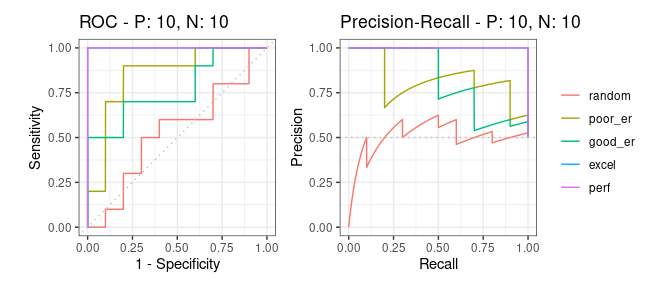#### Example of the as.data.frame function

The as.data.frame function also works with this object.

# Convert mscurves to a data frame
mscurves.df <- as.data.frame(mscurves)

# Use knitr::kable to display the result in a table format
knitr::kable(head(mscurves.df))
x y modname dsid type
0.000 0 random 1 ROC
0.001 0 random 1 ROC
0.002 0 random 1 ROC
0.003 0 random 1 ROC
0.004 0 random 1 ROC
0.005 0 random 1 ROC

## 4. Multiple test sets

The evalmod function calculate performance evaluation for multiple test datasets when different test dataset IDs are specified with the mmdata or the evalmod function.

### Data preparation

There are several ways to create a dataset with the mmdata function for multiple test datasets.

# Specify test dataset IDs names
smmdat1 <- mmdata(scores1, labels2, dsids = c(1, 2))

# Use a sample dataset created by the create_sim_samples function
smmdat2 <- mmdata(samps3[["scores"]], samps3[["labels"]],
dsids = samps3[["dsids"]]
)

### ROC and Precision-Recall calculations

The evalmod function automatically detects multiple test datasets.

# Calculate curves for multiple test datasets and keep all the curves
smcurves <- evalmod(smmdat2, raw_curves = TRUE)

### S3 generics

All the S3 generics are effective for the S3 object generated by this approach.

# Show an average Precision-Recall curve with the 95% confidence bounds
autoplot(smcurves, "PRC", show_cb = TRUE)

# Show raw Precision-Recall curves
autoplot(smcurves, "PRC", show_cb = FALSE)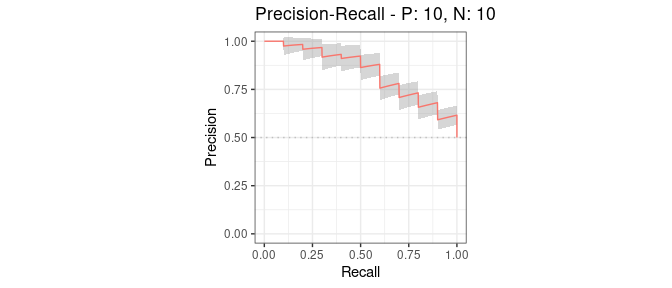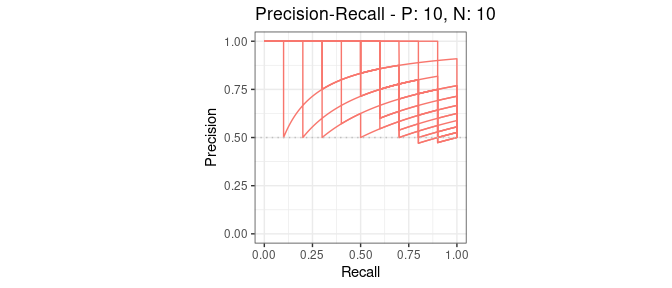#### Example of the as.data.frame function

The as.data.frame function also works with this object.

# Convert smcurves to a data frame
smcurves.df <- as.data.frame(smcurves)

# Use knitr::kable to display the result in a table format
knitr::kable(head(smcurves.df))
x y modname dsid type
0 0.0 m1 1 ROC
0 0.1 m1 1 ROC
0 0.2 m1 1 ROC
0 0.3 m1 1 ROC
0 0.4 m1 1 ROC
0 0.5 m1 1 ROC

## 5. Multiple models and multiple test sets

The evalmod function calculates performance evaluation for multiple models and multiple test datasets when different model names and test dataset IDs are specified with the mmdata or the evalmod function.

### Data preparation

There are several ways to create a dataset with the mmdata function for multiple models and multiple datasets.

# Specify model names and test dataset IDs names
mmmdat1 <- mmdata(scores1, labels2,
modnames = c("mod1", "mod2"),
dsids = c(1, 2)
)

# Use a sample dataset created by the create_sim_samples function
mmmdat2 <- mmdata(samps4[["scores"]], samps4[["labels"]],
modnames = samps4[["modnames"]], dsids = samps4[["dsids"]]
)

### ROC and Precision-Recall calculations

The evalmod function automatically detects multiple models and multiple test datasets.

# Calculate curves for multiple models and multiple test datasets
mmcurves <- evalmod(mmmdat2)

### S3 generics

All the S3 generics are effective for the S3 object generated by this approach.

# Show average Precision-Recall curves
autoplot(mmcurves, "PRC")

# Show average Precision-Recall curves with the 95% confidence bounds
autoplot(mmcurves, "PRC", show_cb = TRUE)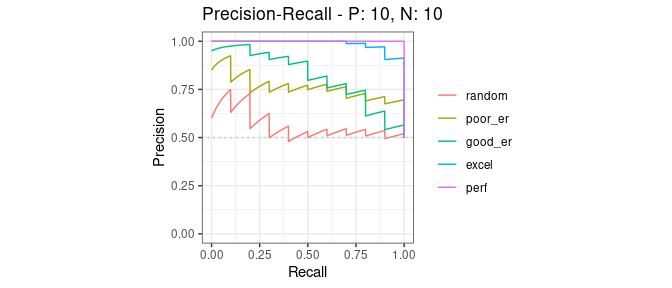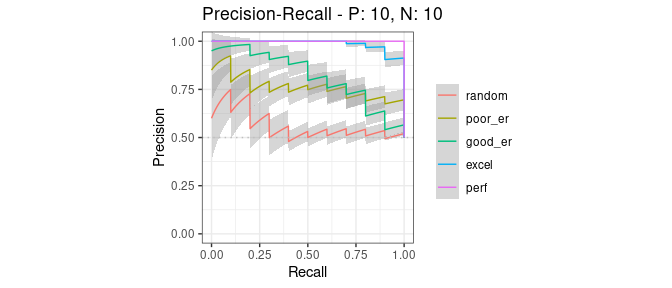#### Example of the as.data.frame function

The as.data.frame function also works with this object.

# Convert smcurves to a data frame
mmcurves.df <- as.data.frame(mmcurves)

# Use knitr::kable to display the result in a table format
knitr::kable(head(mmcurves.df))
x y ymin ymax modname type
0.000 0.000 0.0000000 0.0000000 random ROC
0.000 0.125 0.0665892 0.1834108 random ROC
0.001 0.125 0.0665892 0.1834108 random ROC
0.002 0.125 0.0665892 0.1834108 random ROC
0.003 0.125 0.0665892 0.1834108 random ROC
0.004 0.125 0.0665892 0.1834108 random ROC

## 6. Confidence interval bands

The evalmod function automatically calculates confidence bands when a model contains multiple test sets in provided dataset. Confidence intervals are calculated for additional supporting points, which are specified by the ‘x_bins’ option of the evalmod function.

### Example of confidence bands when x_bins is 2

The dataset smmdat2 contains 20 samples for a single model/classifier.

# Show all curves
smcurves_all <- evalmod(smmdat2, raw_curves = TRUE)
autoplot(smcurves_all)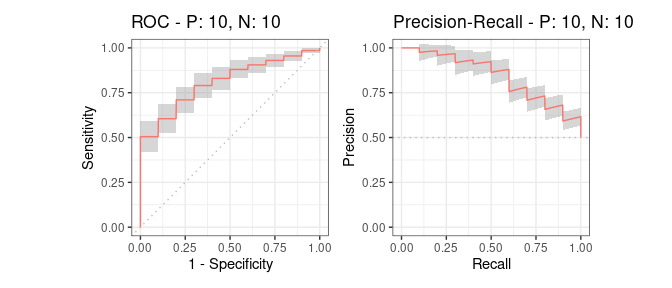Additional supporting points are calculated for x = (0, 0.5, 1.0) when x_bins is set to 2.

# x_bins: 2
smcurves_xb2 <- evalmod(smmdat2, x_bins = 2)
autoplot(smcurves_xb2)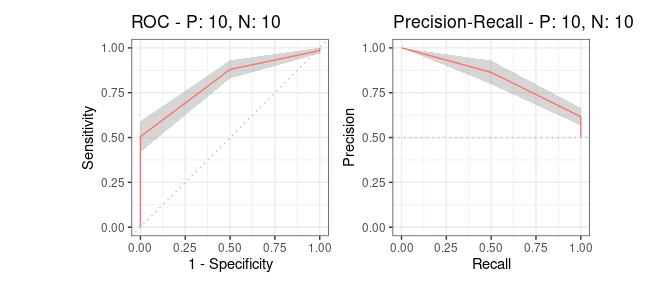### Example of confidence bands when x_bins is 10

Additional supporting points are calculated for x = (0, 0.1, 0.2, 0.3, 0.4, 0.5, 0.6, 0.7, 0.8, 0.9, 1.0) when x_bins is set to 10.

# x_bins: 10
smcurves_xb10 <- evalmod(smmdat2, x_bins = 10)
autoplot(smcurves_xb10)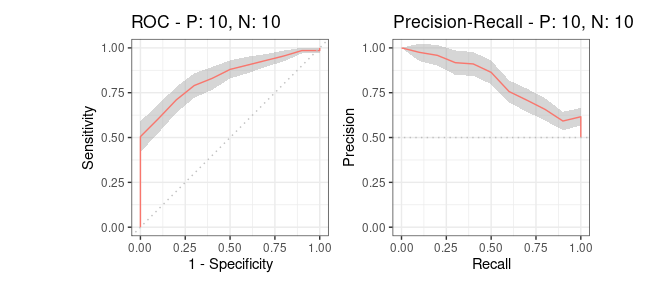### Example of the alpha value

The evalmod function accepts the cb_alpha option to specify the alpha value of the point-wise confidence bounds calculation. For instance, 95% confidence bands are calculated when cb_alpha is 0.05.

# cb_alpha: 0.1 for 90% confidence band
smcurves_cb1 <- evalmod(smmdat2, x_bins = 10, cb_alpha = 0.1)
autoplot(smcurves_cb1)

# cb_alpha: 0.01 for 99% confidence band
smcurves_cb2 <- evalmod(smmdat2, x_bins = 10, cb_alpha = 0.01)
autoplot(smcurves_cb2)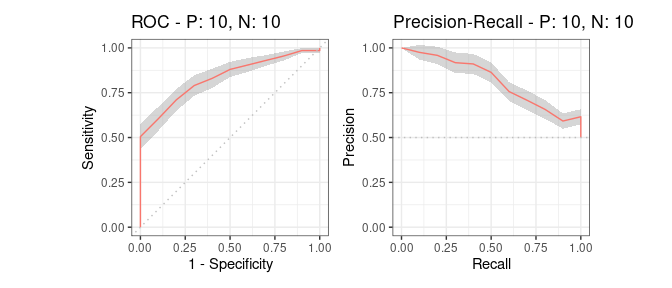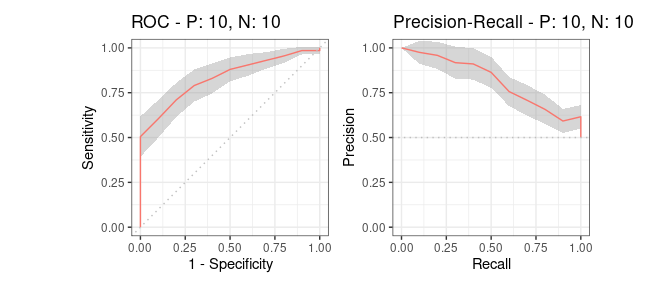## 7. Cross validation

The format_nfold function takes a data frame with scores, label and n-fold columns and convert it to a list for evalmod and mmdata.

#### Example of a data frame with 5-fold data

# Load data
data(M2N50F5)

# Use knitr::kable to display the result in a table format
knitr::kable(head(M2N50F5))
score1 score2 label fold
2.0606025 1.0689227 pos 1
0.3066092 0.1745491 pos 3
1.5597733 -1.5666375 pos 1
-0.6044989 1.1572727 pos 3
-0.2229031 0.6070042 pos 5
-0.7679551 -1.7908147 pos 5

#### Example of the format_nfold function with 5-fold datasets

# Convert data frame to list
nfold_list1 <- format_nfold(
nfold_df = M2N50F5, score_cols = c(1, 2),
lab_col = 3, fold_col = 4
)

# Use column names
nfold_list2 <- format_nfold(
nfold_df = M2N50F5,
score_cols = c("score1", "score2"),
lab_col = "label", fold_col = "fold"
)

# Use the result for evalmod
cvcurves <- evalmod(
scores = nfold_list2$scores, labels = nfold_list2$labels,
modnames = rep(c("m1", "m2"), each = 5),
dsids = rep(1:5, 2)
)
autoplot(cvcurves)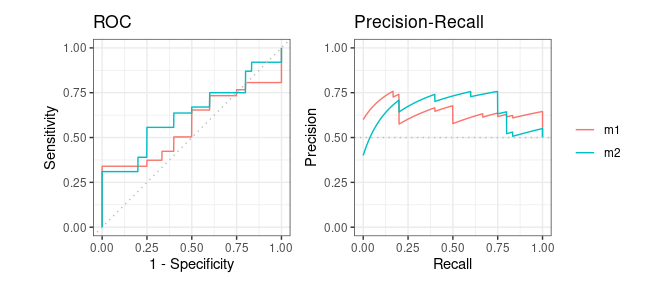### evalmod and mmdata with cross validation datasets

Both evalmod and mmdata function can directly take the arguments of the format_nfold function.

#### Example of evalmod and mmdata with 5-fold data

# mmdata
cvcurves2 <- mmdata(
nfold_df = M2N50F5, score_cols = c(1, 2),
lab_col = 3, fold_col = 4,
modnames = c("m1", "m2"), dsids = 1:5
)

# evalmod
cvcurves3 <- evalmod(
nfold_df = M2N50F5, score_cols = c(1, 2),
lab_col = 3, fold_col = 4,
modnames = c("m1", "m2"), dsids = 1:5
)
autoplot(cvcurves3)## 8. Basic performance measures

The evalmod function also calculates basic evaluation measures - error, accuracy, specificity, sensitivity, and precision.

Measure Description
error Error rate
accuracy Accuracy
specificity Specificity, TNR, 1 - FPR
sensitivity Sensitivity, TPR, Recall
precision Precision, PPV
mcc Matthews correlation coefficient
fscore F-score

### Basic measure calculations

The mode = "basic" option makes the evalmod function calculate the basic evaluation measures instead of performing ROC and Precision-Recall calculations.

# Calculate basic evaluation measures
mmpoins <- evalmod(mmmdat2, mode = "basic")

### S3 generics

All the S3 generics except for auc, part and pauc are effective for the S3 object generated by this approach.

# Show normalized ranks vs. error rate and accuracy
autoplot(mmpoins, c("error", "accuracy"))

# Show normalized ranks vs. specificity, sensitivity, and precision
autoplot(mmpoins, c("specificity", "sensitivity", "precision"))

# Show normalized ranks vs. Matthews correlation coefficient and F-score
autoplot(mmpoins, c("mcc", "fscore"))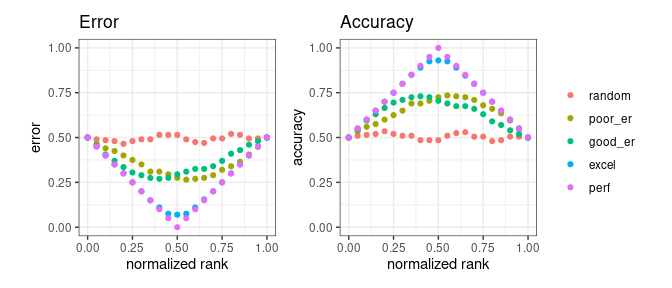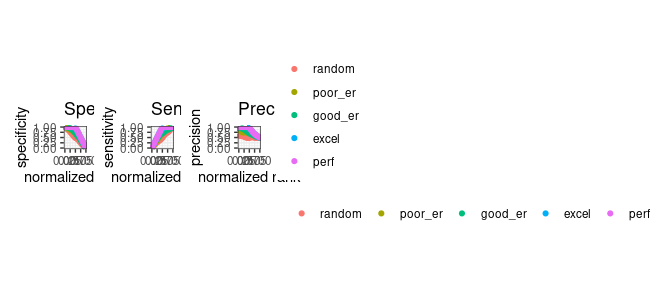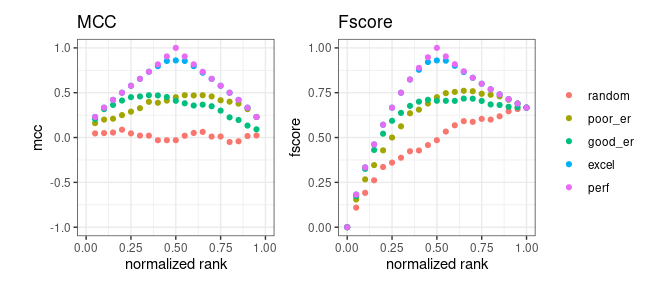#### Normalized ranks and predicted scores

In addition to the basic measures, the autoplot function can plot normalized ranks vs. scores and labels.

# Show normalized ranks vs. scores and labels
autoplot(mmpoins, c("score", "label"))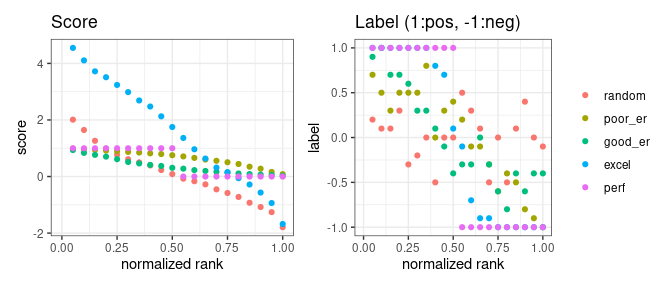#### Example of the as.data.frame function

The as.data.frame function also works for the precrec objects of the basic measures.

# Convert mmpoins to a data frame
mmpoins.df <- as.data.frame(mmpoins)

# Use knitr::kable to display the result in a table format
knitr::kable(head(mmpoins.df))
x y ymin ymax modname type
0.00 NA NA NA random score
0.05 2.0108998 1.8023731 2.2194266 random score
0.10 1.6451511 1.4521817 1.8381204 random score
0.15 1.2638973 1.0932019 1.4345928 random score
0.20 0.9876457 0.8286087 1.1466827 random score
0.25 0.7658987 0.6390489 0.8927484 random score

## 9. Partial AUCs

The part function calculates partial AUCs and standardized partial AUCs of both ROC and precision-recall curves. Standardized pAUCs (spAUCs) are standardized to the score range between 0 and 1.

### partial AUC calculations

It requires an S3 object produced by the evalmod function and uses xlim and ylim to specify the partial area of your choice. The pauc function outputs a data frame with the pAUC scores.

# Calculate ROC and Precision-Recall curves
curves <- evalmod(scores = P10N10$scores, labels = P10N10$labels)

# Calculate partial AUCs
curves.part <- part(curves, xlim = c(0.0, 0.25))

# Retrieve a dataframe of pAUCs
paucs.df <- pauc(curves.part)

# Use knitr::kable to display the result in a table format
knitr::kable(paucs.df)
modnames dsids curvetypes paucs spaucs
m1 1 ROC 0.1006250 0.4025000
m1 1 PRC 0.2345849 0.9383396

### S3 generics

All the S3 generics are effective for the S3 object generated by this approach.

# Show ROC and Precision-Recall curves
autoplot(curves.part)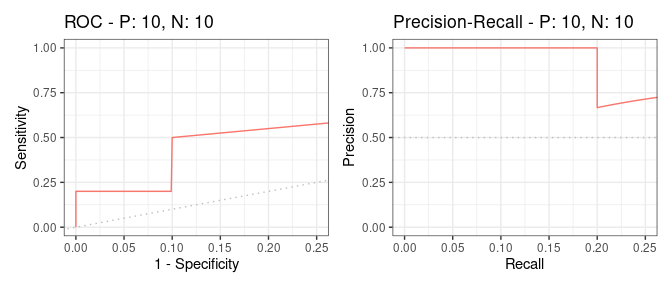## 10. Fast AUC (ROC) calculation

The area under the ROC curve can be calculated from the U statistic, which is the test statistic of the Mann–Whitney U test.

### AUC calculation with the U statistic

The evalmod function calculates AUCs with the U statistic when mode = ‘aucroc’.

# Calculate AUC (ROC)
aucs <- evalmod(scores = P10N10$scores, labels = P10N10$labels, mode = "aucroc")

# Convert to data.frame
aucs.df <- as.data.frame(aucs)

# Use knitr::kable to display the result in a table format
knitr::kable(aucs.df)
modnames dsids aucs ustats
m1 1 0.72 72

## 11. Confidence intervals of AUCs

The auc_ci function calculates confidence intervals of the calculated ROCs by the evalmod function.

### Default CI calculation with normal distribution and alpha=0.05

The auc_ci function calculates CIs for both ROC and precision-recall AUCs. The specified data must contain multiple datasets, such as cross-validation data.

# Calculate CI of AUCs with normal distibution
auc_ci <- auc_ci(smcurves)

# Use knitr::kable to display the result in a table format
knitr::kable(auc_ci)
modnames curvetypes mean error lower_bound upper_bound n
m1 ROC 0.809500 0.0422638 0.7672362 0.8517638 20
m1 PRC 0.842102 0.0360857 0.8060163 0.8781877 20

### CI calculation with a different alpha (0.01)

The auc_ci function accepts a different significance level.

# Calculate CI of AUCs with alpha = 0.01
auc_ci_a <- auc_ci(smcurves, alpha = 0.01)

# Use knitr::kable to display the result in a table format
knitr::kable(auc_ci_a)
modnames curvetypes mean error lower_bound upper_bound n
m1 ROC 0.809500 0.0555440 0.7539560 0.8650440 20
m1 PRC 0.842102 0.0474246 0.7946774 0.8895266 20

### CI calculation with t-distribution

The auc_ci function accepts either normal or t-distribution for CI calculation.

# Calculate CI of AUCs t-distribution
auc_ci_t <- auc_ci(smcurves, dtype = "t")

# Use knitr::kable to display the result in a table format
knitr::kable(auc_ci_t)
modnames curvetypes mean error lower_bound upper_bound n
m1 ROC 0.809500 0.0451330 0.7643670 0.8546330 20
m1 PRC 0.842102 0.0385355 0.8035665 0.8806375 20

## 12. Balanced and imbalanced datasets

It is easy to simulate various scenarios, such as balanced vs. imbalanced datasets, by using the evalmod and create_sim_samples functions.

### Data preparation

# Balanced dataset
samps5 <- create_sim_samples(100, 100, 100, "all")
simmdat1 <- mmdata(samps5[["scores"]], samps5[["labels"]],
modnames = samps5[["modnames"]], dsids = samps5[["dsids"]]
)

# Imbalanced dataset
samps6 <- create_sim_samples(100, 25, 100, "all")
simmdat2 <- mmdata(samps6[["scores"]], samps6[["labels"]],
modnames = samps6[["modnames"]], dsids = samps6[["dsids"]]
)

### ROC and Precision-Recall calculations

The evalmod function automatically detects multiple models and multiple test datasets.

# Balanced dataset
simcurves1 <- evalmod(simmdat1)

# Imbalanced dataset
simcurves2 <- evalmod(simmdat2)

### Balanced vs. imbalanced datasets

ROC plots are unchanged between balanced and imbalanced datasets, whereas Precision-Recall plots show a clear difference between them. See our article or website for potential pitfalls by using ROC plots with imbalanced datasets.

# Balanced dataset
autoplot(simcurves1)

# Imbalanced dataset
autoplot(simcurves2)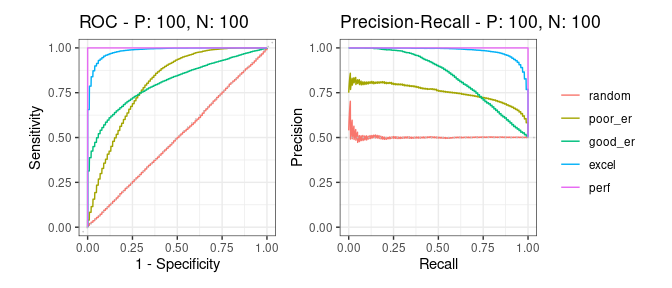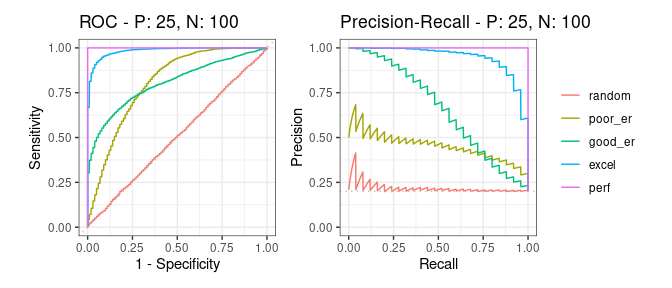## 13. Citation

Precrec: fast and accurate precision-recall and ROC curve calculations in R

Takaya Saito; Marc Rehmsmeier

Bioinformatics 2017; 33 (1): 145-147.